heat control  r71
lcdisp.h File Reference
`#include "types.h"`
Include dependency graph for lcdisp.h: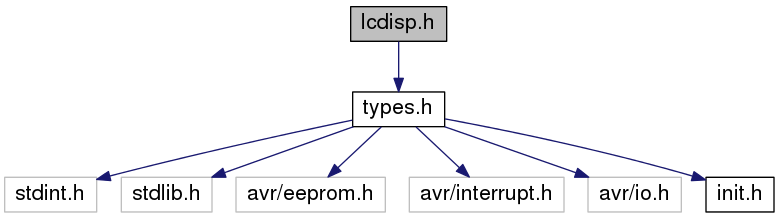This graph shows which files directly or indirectly include this file: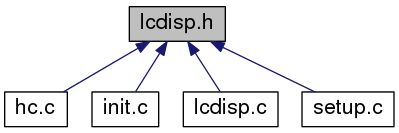Go to the source code of this file.

struct  LCD_POS

struct  MEASVAL

## Macros

#define USE_LCD   16

#define NUM_BASE   10

#define ALNUM_BASE   0x30

#define NUM_LEN   6

#define NUM_SDG   5

#define VALUE_LENGTH   8

#define BR_DBDP   3

#define DF_DECPT   '.'

#define NAME_LENGTH   1

#define FW_REV   "R071"

## Typedefs

typedef struct LCD_POS LCD_POS

typedef struct MEASVAL MEASVAL

typedef struct SETUP_ITEM SETUP_ITEM

## Functions

void LcdInit (void)
display initialization Alphanumeric LCD initialization Connections are specified in the Project|Configure|C Compiler|Libraries|Alphanumeric LCD menu: RS - PORTA Bit 0 RD - PORTA Bit 1 EN - PORTA Bit 2 D4 - PORTA Bit 4 D5 - PORTA Bit 5 D6 - PORTA Bit 6 D7 - PORTA Bit 7 Characters/line: 16 More...

void WriteMeasVal (MEASVAL *mv)
put a measurement value on lcd source value is being output as fixed decimal point number More...

void WriteValue (MEASVAL *mv)
write the payload value itself onto lcd while considering the data type More...

void WriteSetupValue (SETUP_ITEM *si, UCHAR allow, UCHAR id)
put a setup item on screen, which consist of a name and a value spread over two lines More...

void WriteSetupGroup (UCHAR grp)

void WriteCtrlMode (UCHAR name, UCHAR *cmName)

void ntofa (UINT val, UCHAR *buf, DATTYPE dt)
convert integer value to fixed decimal point string buffer must have at least a size of 8 bytes allocated More...

UINT _exp10 (UCHAR n)

void Welcome (void)
put startup info on screen
More...

## Macro Definition Documentation

 #define ALNUM_BASE   0x30

alphanumeric base

Definition at line 18 of file lcdisp.h.

Referenced by ntofa().

 #define BR_DBDP   3

number of digits before decimal point

Definition at line 34 of file lcdisp.h.

Referenced by ntofa().

 #define DF_DECPT   '.'

default decimal point

Definition at line 38 of file lcdisp.h.

Referenced by ntofa().

 #define FW_REV   "R071"

firmware revision

Definition at line 46 of file lcdisp.h.

Referenced by Welcome().

 #define NAME_LENGTH   1

length of name in a measurement value

Definition at line 42 of file lcdisp.h.

 #define NUM_BASE   10

decimal number base

Definition at line 14 of file lcdisp.h.

Referenced by _exp10().

 #define NUM_LEN   6

length of a mv number (without sign and decimal point)

Definition at line 22 of file lcdisp.h.

Referenced by ntofa().

 #define NUM_SDG   5

number of significant digits of a mv value (MAX of UINT)

Definition at line 26 of file lcdisp.h.

Referenced by _exp10().

 #define USE_LCD   16

number of columns of used lcd

Definition at line 10 of file lcdisp.h.

 #define VALUE_LENGTH   8

length of mv value (including sign, decimal point and terminating null byte)

Definition at line 30 of file lcdisp.h.

Referenced by ntofa(), and WriteValue().

## Typedef Documentation

 typedef struct LCD_POS LCD_POS

definition of a position and the alignment of a string to put on LCD

 typedef struct MEASVAL MEASVAL

definition of a measurement value to put on LCD

 typedef struct SETUP_ITEM SETUP_ITEM

Definition at line 78 of file lcdisp.h.

## Function Documentation

 UINT _exp10 ( UCHAR n )

calculate the exponential to base 10

Parameters
 n number to calculate the exponential for
Returns
result of exponential to base 10

Definition at line 201 of file lcdisp.c.

References NUM_BASE, NUM_SDG, UCHAR, and UINT.

Referenced by ntofa().

Here is the caller graph for this function: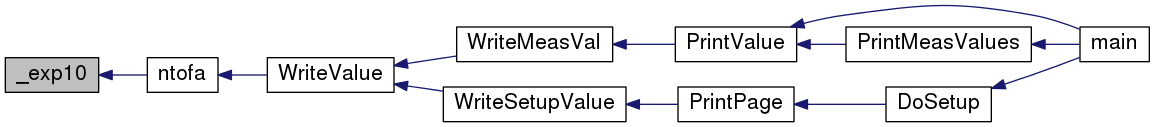void LcdInit ( void )

display initialization Alphanumeric LCD initialization Connections are specified in the Project|Configure|C Compiler|Libraries|Alphanumeric LCD menu: RS - PORTA Bit 0 RD - PORTA Bit 1 EN - PORTA Bit 2 D4 - PORTA Bit 4 D5 - PORTA Bit 5 D6 - PORTA Bit 6 D7 - PORTA Bit 7 Characters/line: 16

Definition at line 23 of file lcdisp.c.

References LCDclr(), LCDinit(), and Welcome().

Referenced by Init().

Here is the call graph for this function: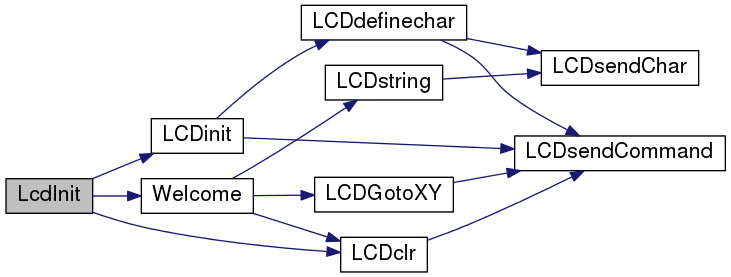Here is the caller graph for this function: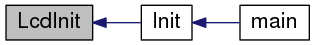void ntofa ( UINT val, UCHAR * buf, DATTYPE dt )

convert integer value to fixed decimal point string buffer must have at least a size of 8 bytes allocated

Parameters
 val value being converted buf buffer for the conversion result dt data type to convert to

Definition at line 133 of file lcdisp.c.

References _exp10(), ALNUM_BASE, BR_DBDP, DF_DECPT, DT_CHAR, DT_INT, DT_TIME, DT_UCHAR, NUM_LEN, UCHAR, UINT, and VALUE_LENGTH.

Referenced by WriteValue().

Here is the call graph for this function: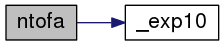Here is the caller graph for this function: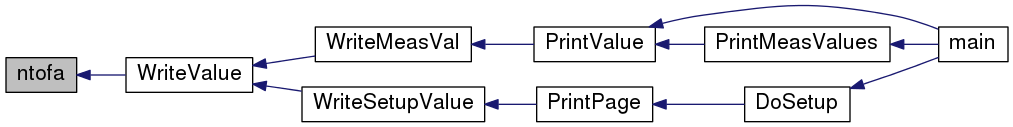void Welcome ( void )

put startup info on screen

• project name
• revision or version number
• vendor's name

Definition at line 225 of file lcdisp.c.

References FW_REV, LCDclr(), LCDGotoXY(), LCDstring(), and UCHAR.

Referenced by LcdInit().

Here is the call graph for this function:Here is the caller graph for this function:void WriteCtrlMode ( UCHAR name, UCHAR * cmName )

puts the control mode onto screen and gives it a name

Parameters
 name of the control mode (of meas value) cmName hrf control mode

Definition at line 117 of file lcdisp.c.

References LCDGotoXY(), LCDsendChar(), LCDstring(), and UCHAR.

Referenced by main().

Here is the call graph for this function: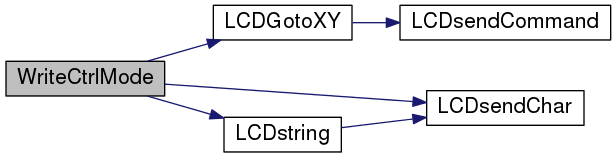Here is the caller graph for this function: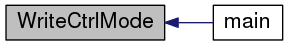void WriteMeasVal ( MEASVAL * mv )

put a measurement value on lcd source value is being output as fixed decimal point number

Parameters
 mv measurement value to extract values to put on screen from

Definition at line 34 of file lcdisp.c.

Referenced by PrintValue().

Here is the call graph for this function:Here is the caller graph for this function: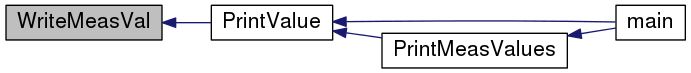void WriteSetupGroup ( UCHAR grp )

puts the hrf setup group onto screen

Parameters
 grp encoded group to resolve to hrf and print

Definition at line 95 of file lcdisp.c.

References LCDGotoXY(), LCDstring(), POS_GRP_START, SG_CTRL, SG_LIMIT, SG_MEAS, and UCHAR.

Referenced by DoSetup().

Here is the call graph for this function:Here is the caller graph for this function: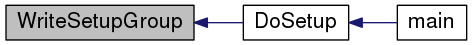void WriteSetupValue ( SETUP_ITEM * si, UCHAR allow, UCHAR id )

put a setup item on screen, which consist of a name and a value spread over two lines

Parameters
 si setup item to print allow flag to allow (TRUE) or disallow (FALSE) writing - this reflects the currently selected item to the user by flashing id of the position in data array, used for position determination on screen

Definition at line 72 of file lcdisp.c.

Referenced by PrintPage().

Here is the call graph for this function: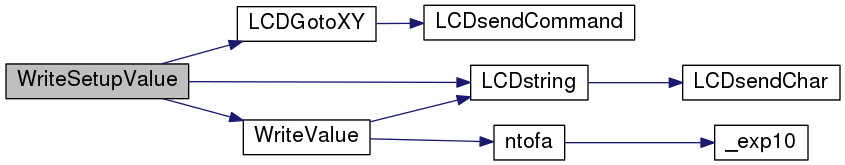Here is the caller graph for this function: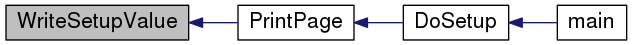void WriteValue ( MEASVAL * mv )

write the payload value itself onto lcd while considering the data type

Parameters
 mv encapsulated measurement value to put on screen

Definition at line 44 of file lcdisp.c.

References MEASVAL::dt, DT_CHAR, DT_FLOAT, DT_INT, DT_TIME, DT_UCHAR, DT_UINT, LCDstring(), ntofa(), UCHAR, MEASVAL::value, and VALUE_LENGTH.

Referenced by WriteMeasVal(), and WriteSetupValue().

Here is the call graph for this function: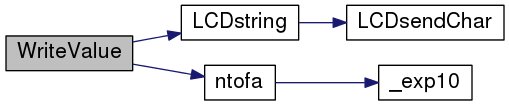Here is the caller graph for this function: